Mobile QR Code1. (Faculty of Information Technology, Electric Power University, 235 Hoang Quoc Viet Rd, Hanoi, Vietnam {anhdn, tungnk}@epu.edu.vn )
2. (School of Information & Technology, Hanoi University of Science and Technology, 1 Dai Co Viet Rd, Hanoi, Vietnam minh.nh194120@sis.hust.edu.vn )

Clusters, Building-energy consumption, Gradient boosting, k-Means, Agglomerative clustering

1. Introduction

It is increasingly common for energy organizations to gather and study energy consumption for business management. Coordinating the tasks to forecast usage by consumers requires reliability and precision. During the last four decades, numerous efforts have been directed at developing smart sensors attached to electricity meters, and making data on energy consumption available in practice. The promise is that computer-assisted analysis of such large amounts of data will enhance screening dimensions, reduce the problem of observer dependency, and reinforce diagnostic certainty in residential energy usage.

In particular, the demand for electricity in Vietnam in 2021 was forecast by Electricity Company of Vietnam  based on a basic plan and an intensive plan. The basic plan consists of commercial electricity demand at 235.2 billion kWh, corresponding to electricity production capable of providing 267.9 billion kWh. The intensive plan covers 236.97 billion kWh in electricity consumption while the production capability is expected to be 269.9 billion kWh.

Note that energy usage in high-rise buildings with more than 10 floors has received considerable attention based on very high rates at about 40% of total energy consumption in the world, according to one United Nations Environment Program report .

The consumption rate for high-rise buildings in Vietnam is 35% to 40% of total energy consumption in the country. According to an Emporis report , there are 1443 high-rise buildings of which 1384 are occupied, 46 are under construction, and 11 are in the planning stages. We note that not all the buildings have smart meters to measure power consumption once a day. There are buildings where the data are collected manually each month. At present, the data allow analyzing and making predictions for future power consumption. The analysis, conceptually, appears to be generally well regarded for optimizing power generation and balancing the distribution grid, but in reality is proving difficult to fully adopt. This difficulty comes down to a combination of necessity in maintaining a smart meter system, and a lack of data analysis. Taken together, we put forward the argument that building-energy consumption is an interdisciplinary topic that would greatly benefit from data mining and potential machine learning research.

In this article, we look at an application-driven case study. Informally, we show how data mining techniques can be extended to handle building energy-consumption data, which may be regarded as an estimate of future power consumption associated with building patterns.

Our main contributions are to define energy consumption patterns in the apartments of a residential building; to prove that the patterns are tractable for weighted clustering; to apply a k-Means and agglomerative clustering algorithm to solve grouping problems with apartment-dependent energy consumption constraints; to implement gradient boosting for predicting energy consumption for each group of apartments and then grouping them to make forecasts available for the whole building; to provide a comprehensive experimental evaluation of our method; and to show how the performance of our method compares very favorably with an approach that uses gradient boosting.

Our experimental evaluation shows that the method enjoys substantial predominance over traditional methods for implementing clustering analysis. The proposed method is fit to deal far better with five clusters for our particular selected dataset. In the next section, we present the data mining method, having reviewed relevant concepts from the literature.

2. Related Work

We first review methods aimed at electricity-consumption forecasting for residential buildings in (I). Then, we discuss works focusing on gradient boosting and clustering analysis in (II).

$\textbf{(I)}$ It is clear that accurate energy consumption prediction is more important to better avoid energy waste and to ameliorate the quality and effectiveness of energy systems. Specifically, machine learning methods can be implemented to discover data patterns and to predict how an electricity network works. An important note is that every consumer is different, and they all behave in a dissimilar manner. Hence, Paulo et al.  presented a comparison report on how machine learning methods are applied to building-energy consumption.

Four methods, Linear Regression, the Decision Forest, the Boosted Decision Tree, and the Artificial Neural Network (ANN), were addressed in experiments with the same real dataset collected over a period of 150 days in two houses in Iceland.

González-Briones et al.  demonstrated variations in the prediction of energy consumption by using k-Nearest Neighbors, Linear Regression, Random Forest, Support Vector Regression, and the Decision Tree. Thus, the dataset used for experiments was taken from a shoe store located in Salamanca, Spain, showing the daily electricity consumption of a dwelling with two people living in it. Klemenjak et al.  conducted an analysis of more than a dozen datasets to offer recommendations for electricity-consumption data collection, storage, and provision. Based upon the recommendations, datasets with increased usability and comparability can be created.

Zhang  dealt with anomalous consumption detection based on data mining techniques. The proposed method focuses on each formula to evaluate anomalies in each different time scale, without using any machine learning algorithms. The dataset was per-household basics, which can be expanded, but it is necessary to change formulas to assess consumption-based anomalies.

These papers addressed forecasting of residential electricity consumption. Although different databases were used for the studies, there are cases where a lack of data can be observed. The time-series forecast is based principally on the provided data, and a lack of data could lead to wrong predictions. We address this problem in our case study with a database in which data were lost for some intervals. In particular, our solution will include applicable data mining techniques for the case.

$\textbf{(II)}$ Vantuch et al.  showed that forecasting accuracy decreases with an increase in the time scale due to the impossibility of using all variables. The authors processed Support Vector Regression, Random Forest Regression, eXtreme Gradient Boosting (XGBoost), and the Flexible Neural Tree on a dataset obtained from Murcia University buildings in Spain spanning nearly one year. The objective was to predict power consumption for those buildings in the subsequent few hours. The data were collected at a 15-minute sampling rate. The best forecast results were achieved by using XGBoost.

To establish a model for energy consumption in a residential house, Ashouri et al.  created a database from 76 buildings in Japan from 2002 to 2004 consisting of eight types of electrical equipment. It can be seen that the relation between climate conditions, building characteristics, building services, and operations were analyzed using clustering analysis and ANN models. Then, recommendations on the use of energy for the inhabitants of a building can be generated from the ANN. Xu and Chen  conducted a case study to find anomalies in data from residential buildings. The solution outlined in the paper is a combination of the Recurrent Neural Network (RNN) and quantile regression. However, this is a short paper missing details on the experimental results.

Clustering-based analysis was addressed by Ullah et al.  in order to categorize consumers’ electricity usage into different levels. This work used a deep auto-encoder to transfer low-dimensional energy consumption data into high-level representations. Then, an adaptive self-organizing map-clustering algorithm with statistical analysis determined the levels of electricity consumption.

In principle, XGBoost, the ANN, and the RNN were implemented in the above-mentioned methods to resolve forecasting. However, due to the discontinuities in the time-series data, the accuracy of prediction can drop. To allow the deep learning method to work with insufficient data we propose applying both feature engineering and XGBoost involving proper clustering. In the following, our method (with details) is described.

3. The Method

We follow the paradigm of Bayesian time-series analysis introduced by Barber et al. . This involves the construction of a probability model for apartment energy consumption. For convenience, the following notations are used in describing the model:

$i$ - apartment, $i~ =1\colon n$

$x_{i}$ - energy condition of apartment $i$

$t=1\colon T$ - a time-series

$c_{j}$ - clusters, $j~ =1\colon k$

$e_{t}\left(x_{i}\right)$ - energy consumption of an apartment $i$

$e_{t}~ =~ mean_{j=1\colon n}e_{t}\left(x_{i}\right)$ - averaged consumption of apartments

The functional elements of our method are described in two main parts: covering gradient boosting in (I) and clustering analysis in (II).

$\textbf{(I)}$ To express the learning process in our method, we use Bayes’ Rule  that traces out conditional probability for class $c$ and sample $s$:

(1)
$p\left(c,s\right)=p\left(c|s\right)p\left(s\right)$

A common way of writing a probabilistic model of a time series for energy consumption, $e_{1\colon T}~ =e_{1},e_{2},\ldots e_{T},$ expresses the statement of a joint distribution $p\left(e_{1\colon T}\right)$.

In practice, however, identifying all independent entries of $p\left(e_{1\colon T}\right)$ is impracticable without making some statistical independence assumptions. So, for a time series of more than a few steps, it is necessary to introduce simplifications for traceability. Thus, we replace $c$ with $e_{T}$ and $s$ with $e_{1\colon T-1}$ in (1); reordering, we have

(2)
$p\left(e_{T}|e_{1\colon T-1}\right)=p\left(e_{T},e_{1\colon T-1}\right)/p\left(e_{1\colon T-1}\right)$

Furthermore, we can break down $p\left(e_{1\colon T-1}\right)$ as follows:

(3)
$p\left(e_{1\colon T-1}\right)=p\left(e_{T-1}|e_{1\colon T-2}\right)/p\left(e_{1\colon T-2}\right)$

By continuing the exercise, the joint distribution can be seen as follows:

(4)
$p\left(e_{1\colon T}\right)=\prod _{t=1}^{T}p\left(e_{t}|e_{t\colon t-1}\right)$

The above factorization is consistent, based on the causal nature of time (where each factor expresses a generative model of a variable conditioned on its past) by plugging in conditional independence to release the variables in each factor-conditioning set. Note that by imposing $p\left(e_{t}|e_{1\colon t-1}\right)=p(c|e_{t-m\colon t-1})$, we can derive the $m$th-order Markov model, which is of fundamental importance in many time-series models . In an$~ m$th\hbox{-}order Markov model, the joint distribution factorizes as

(5)
$p\left(e_{1\colon T}\right)=\prod _{t=1}^{T}p\left(e_{t-m\colon t-1}\right)$

The auto-regressive (AR) model is a Markov model of continuous scalar observations . For an $m$th-order AR model, we assume a statement of $e_{t}$ is a noisy linear combination of the previous $m$ observations:

(6)
$e_{t}=a_{1}a_{t-1}+a_{2}a_{t-2}+\ldots +a_{m}a_{t-m}+\varepsilon _{t}$

where $a_{1\colon m}$ represents coefficients, and $\varepsilon _{t}$ is independent noise that is assumed to be zero-mean Gaussian with variance $r$. In this step, a generative form for the prediction model with Gaussian noise is represented by

(7)
$p\left(e_{1\colon T}|e_{1\colon m}\right)=\prod _{t=m+1}^{T}p\left(e_{t}|e_{t-m\colon t-1}\right)$

where energy consumption value $e_{t}$ is a function of $m$ previous moments:

(8)
$e_{t}=f\left(e_{t-m,.},e_{t-1}\right)$

From (7) it is possible to show that

(9)
$p\left(e_{t}|e_{t-m\colon t-1}\right)=N\left(f\left(e_{t-m},.,e_{t-1}\right),r\right)$

Using a gradient boosting method, the learning objective of Gradient Tree Boosting [15,16] is minimization of the error between predicted value $e_{t}$ and actual value $\hat{e}_{t}$. This minimization is formulated by the following equation:

(10)
$L=l(e_{t}$, $\hat{e}_{t})+\lambda$

where $l$ is the loss function that calculates the prediction error, and $\lambda$ is a function that regularizes the learning task to control overfitting.

$\textbf{(II)}$ Now, we get a closer look at the data level of the apartments; observation of the energy consumption in a building, $e_{t}$, is calculated by averaging the consumption of the apartments:

(11)
$e_{t}=mean_{j=1\colon n}e_{t}\left(x_{i}\right)$

where $x$ denotes apartment conditions for energy consumption, while $e$ is the consumption value observed at time $t.$ Fig. 1(a) shows the relationship between $x,e$, and$~ t$.

To improve smoothness, we apply clustering to the apartment conditions for energy consumption $x$ by introducing cluster $c$ for each apartment, as seen in Fig. 1(b). It is well known that k-Means  is a method for clustering a dataset, $X=x_{1},x_{2},\ldots x_{N}$, of $N$ unlabeled data points into $K~$clusters, where $K$ is specified by the user. In our study of energy consumption based on (9), the objective of the k-Means algorithm is to minimize the following cost function:

(12)
$V_{t-m\colon t-1}=\sum _{i=1}^{K}\sum _{x_{j}\in C_{i}}\left(e_{t-m\colon t-1}\left(x_{j}\right)-\mu _{i}\right)^{2}$

where $C_{i}$ denotes a cluster, and $\mu _{i}$ is the center of cluster $C_{i}$. The minimization of $V_{t-m\colon t-1}$ in (12) allows us to assign cluster $c$ for each apartment $x_{j}$ using consumption conditions during the past $\left(t-m\colon t-1\right)\colon$

(13)
$c_{t-m\colon t-1}\left(x_{j}\right)=\textit{argmi}n_{i=1\colon K}\left(e_{t-m\colon t-1}\left(x_{j}\right)-\mu _{i}\right)^{2}$

where the $c_{i}$ is the cluster assigned for apartment $x_{j}$, and $\mu _{i}$ is the relevant cluster center.

Given the energy consumption for each apartment from (13), implementation of (11) permits us to obtain the average energy consumption for the whole building in the past:

(14)
$e_{t-m\colon t-1}\left(c_{i}\right)=mean_{x\in {C_{i}}}c_{t-m\colon t-1}\left(x_{j}\right)$

It should be apparent that general prediction formula (7) can be rewritten for each cluster as follows:

(15)
$p\left(e_{1\colon T}\left(c_{i}\right)|e_{1\colon m}\left(c_{i}\right)\right)=\prod _{t=m+1}^{T}p\left(e_{t}\left(c_{i}\right)|e_{t-m\colon t-1}\left(c_{i}\right)\right)$

Similarly, the prediction condition based on $m$ previous moments (9) has its specific form applied for a cluster:

(16)
$p\left(e_{1\colon T}\left(c_{i}\right)|e_{1\colon m}\left(c_{i}\right)\right)=N\left(\sum _{i=1}^{m}a_{i}e_{t-i}\left(c_{i}\right),r\right)$

Finally, the prediction of energy consumption for the building is achieved by getting a weighted average of the above prediction for clusters:

(17)
$p\left(e_{t}|e_{t-m\colon t-1}\right)=\sum _{i=1}^{m}w\left(c_{i}\right)p\left(e_{t}\left(c_{i}\right)|e_{t-m\colon t-1}\left(c_{i}\right)\right)$

where $w\left(c_{i}\right)$ is the weight of cluster $c_{i}$. The weight is proportional to the number of elements $\left(\textit{numel}\right)$ assigned to the cluster:

(18)
$w\left(c_{i}\right)=\frac{\textit{numel}\left(c_{i}\right)}{\sum _{i=1}^{m}\textit{numel}\left(c_{i}\right)}$

While we have shown that the method is based on gradient boosting and clustering, it is also possible to use some metrics for performance estimation.

By using $e_{i}$ and $\hat{e}_{i}$ to denote prediction value and actual value, respectively, the Mean Squared Error, Mean Absolute Error, Root Mean Squared Error, and Mean Absolute Percentage Error are defined by Eqs. (19) to (22):

(19)
$MSE=\frac{1}{n}\sum _{i=1}^{n}\left(e_{i}-\hat{e}_{i}\right)^{2}$
(20)
$MAE=\frac{1}{n}\sum _{i=1}^{n}\left| e_{i}-\hat{e}_{i}\right|$
(21)
$RMSE=\sqrt{MSE}$
(22)
$MAPE=\frac{1}{n}\sum _{i=1}^{n}\left| \frac{e_{i}-\hat{e}_{i}}{\hat{e}_{i}}\right|$
Fig. 1. Relationships in energy consumption models. a Energy consumption $e$ by time $t$ depends on the consumption conditions of apartment $x$. b-Energy consumption $e$ by time $t$ depends on the consumption conditions of cluster $c$ of apartment $x$.}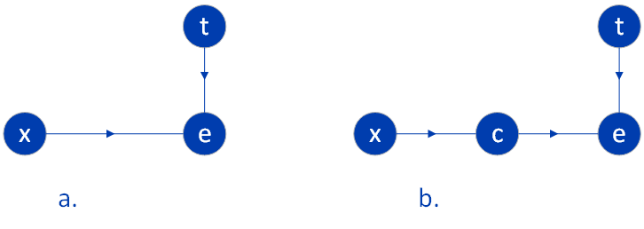4. Experimental Results

Keys to effective time-series prediction are data analysis and the selection of a suitable learning method. We conducted experiments in three steps: data analysis, gradient boosting, and data clustering.

4.1 Data Analysis

The proposed method was evaluated on an electricity-consumption dataset collected from apartment units in buildings in Hanoi, Vietnam. The data were gathered by smart sensors that allowed recording energy consumption in wattage for apartments in buildings. The data cover more than 500 apartments and offices from three buildings over two years (from September 2014 to December 2016) providing three basic features: $ID$ (indicating apartment units), $pkw$ (for wattage), and of course, the index field of date and time.

Note that there may be apartments and offices in the same building. Despite having a huge number of data points, the data are not consecutive and were split into intervals that can cover a few months. This leads to the difficulty of discovering a data trend as it intuitively should be, and exploring data seasonality, which is often a significant factor in power consumption. Since gradient boosting (8-12) is a decision tree-based algorithm, we designed additional features extracted from the original date-time feature in order to help the algorithm find patterns in the data.

The extracted features are day of the week, the quarter, the month, the year, the day of the year, the day of the month, and the week of the year, which are commonly used to handle time-series problems. By checking the data distribution by apartment, we found that the distribution was uneven. Hence, the total amount of electricity consumption cannot be gathered from the recorded wattage.

However, we chose averaging the wattage for further analysis and prediction. Fig. 2 demonstrates the energy consumption distribution of the building based on days of the week and hours of the day in the form of a heat map, where the colors show the levels of consumption.

It is clear that the levels of energy consumption for work days are mostly the same, whereas they decrease slightly for weekends. During the day, a high level of energy consumption is observed for the hours from 7 am to 10 am and from 1 pm to 4 pm in the afternoon. The consumption distribution completely fits Electricity of Vietnam’s consumption pricing list , which is split into sections for days and hours.

To implement gradient boosting for time-series apartment energy consumption based on Eqs. (8) to (12) for this case study, we employed the Extreme Gradient Boosting (XGBoost) package developed by Chen et al. . This is an open-source software library providing a regularizing gradient boosting framework.

The package often performs more efficiently than other algorithms, such as ARIMA  or PROPHET , when there is a lack of data because it does not need to discover cycle patterns. The issue is found in our data, and that is why XGBoost was our choice for the time-series solution.

In order to demonstrate the proposed method, we started with the original XGBoost to get the initial performance. By applying the metrics in Eqs. (19) to (22), the results are as follows:

$\textbf{XGBoost}$

MSE: 0.007421028512366852

MAE: 0.05360403479255469

RMSE: 0.0861453917070835

MAPE: 20.385228594547005

Fig. 3 shows the actual energy consumption in blue, while the prediction is in red. Note that the model seems to be confused with huge swings of data, and consequently, it becomes too conservative and has not learned anything.

Fig. 2. Energy consumption distribution of the building based on days of the week and hours of the day.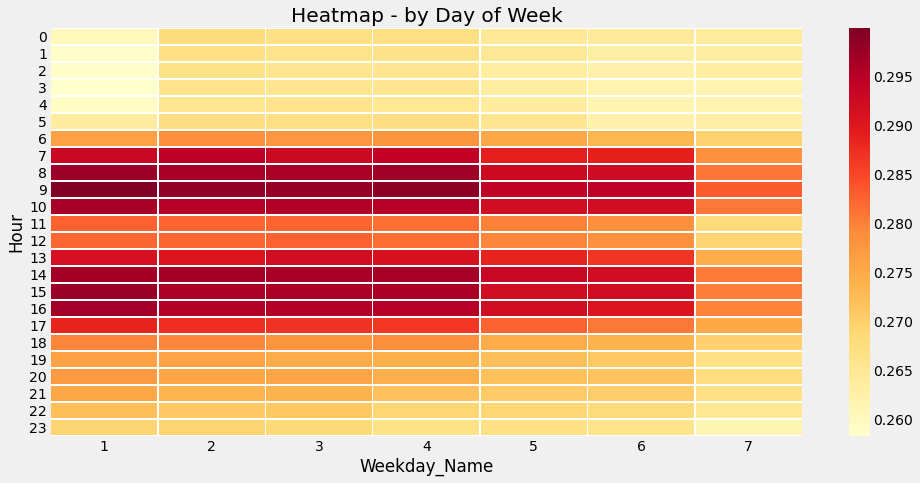Fig. 3. Prediction by the original XGBoost showing huge swings of data in our case study. Blue presents actual values, and red indicates the predicted values.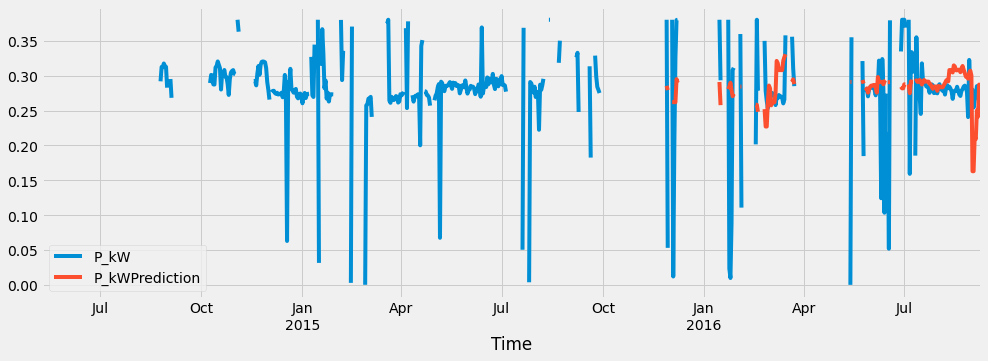4.3 Data Clustering

Acknowledging the fact that the dataset contains different $IDs$ indicating different apartments and offices that are associated with different energy consumption conditions, applying data clustering with formulas (12) to (18) for these $IDs$ could improve prediction performance.

We created an additional 170 features, including the $pkw$ average for each hour in a day, each day in a week, and the total mean and standard deviation. The aim is to learn differentiations that may exist in consumption conditions between apartments or between apartments and offices. Therefore, we applied clustering techniques to categorize the dataset into groups by using original features and newly extracted features.

In the following, two cluster algorithms have been used: k-Means clustering and agglomerative clustering. What is important is that the task of gradient boosting prediction is conducted separately for each group of apartments based on Eqs. (12) to (16), and is then summarized with Eqs. (17) and (18). Consider XGBoost with support for clustering the dataset by k-Means. We can see performance improvement through results reported with $k=18$:

$\textbf{k-Means Clustering & XGBoost}$

MSE: 0.001961885122344051

MAE: 0.030668140386704996

RMSE: 0.04429317241228101

MAPE: 11.192344669596173

With k-Means, the predictions in Fig. 4 (in red) show short distances from the actual values in blue. Compared with Fig. 3, there is a noticeable improvement in the predictions.

As can be seen, the performance metrics for predictions using agglomerative clustering and XGBoost are ameliorated, compared to using only XGBoost:

$\textbf{Agglomerative Clustering & XGBoost}$

MSE: 0.002539348382033498

MAE: 0.031479287301019958

RMSE: 0.05039194759119257

MAPE: 13.59158428571727

In the experiment results shown in Fig. 5, XGBoost was implemented with the assistance of agglomerative clustering ($k~ =~ 22$). Prior to clustering, the predictions (in red) closely follow the blue actual values. Although more computationally expensive, it provides a smooth curve, making it a preferable candidate for evaluating the usefulness of the predictions.

Table 1 presents an MAE report from the experiments that were conducted to evaluate the sensitivity of parameter $k$ for clustering data by using the k-Means and agglomerative clustering methods. The first case with $k=1$ is implementation of XGBoost without any clustering, where the scores are printed in bold.

The second case is when we applied clustering with two clusters, $k~ =~ 2$, which changes the MAE value from 0.0536 to 0.0433 for k-Means clustering; from 0.0536 to 0.0426 for agglomerative clustering. Prior to the value of parameter $k,$ MAE changed for both clustering methods. The experiments were applied to 29 values of $k$. Fig. 6 shows the model of $k$ variations for the k-Means clustering. We note a sharp drop in MAE results when $k$ runs from 1 to 5. Then the speed is observed to slow down before starting to fluctuate when $k$ reaches 15.

The event can be interpreted as either overfitting or the amount of data points of a single cluster is too small. No other significant drops were detected for the remaining 14 blocks of $k$.

Fig. 7 shows an accentuated drop in performance metrics from MSE, MAE, and RMSE for agglomerative clustering in the clustering data based on the five blocks with $k~ =~ 2\colon 5$ before learning the time-series. Fig. 7 also shows no other remarkable drops for the remaining 24 blocks of $k.$

Table 2 displays the performance of the implemented methods for comparison. The results from XGBoost are presented in the second row with MSE of 0.007. A combination of agglomerative clustering with $k=22$ and XGBoost reduces the error metric of MSE to 0.002. In the last row, application of k-Means ($k=18$) with XGBoost achieved an MSE of 0.001.

For other metrics covering MAE, RMSE, and MAPE, a distinct enhancement was observed for both clustering methods.

We note the lowest errors were delivered by the combination of k-Means and XGBoost, where the best scores are underlined.

We suspect better performance could be achieved by sampling more of the data points to fill lost time intervals. However, our overall focus is more on the differentiation of consumption conditions, so we do not believe this would be useful for our primary goals in the time-series analysis.

Fig. 4. XGBoost with support from k-Means clustering when $k=18$. Actual energy consumption is displayed in blue, whereas predictions are in red.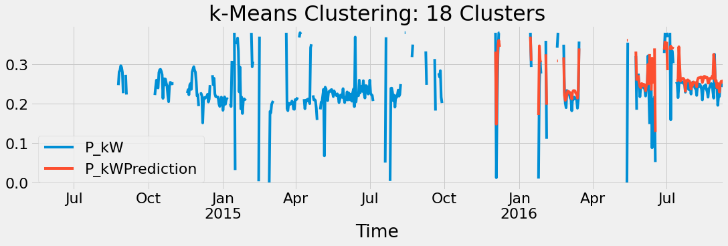Fig. 5. XGBoost with the support of agglomerative clustering when $k~ =~ 22$. Actual energy consumption is displayed in blue, whereas predictions are in red.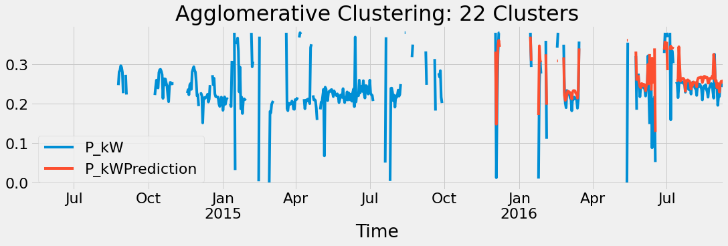Fig. 6. Performance of XGBoost and k-Means clustering by changing the $k~$parameter: MSE-yellow, MAE-red, RMSE-blue.Fig. 7. Performance of XGBoost and Agglomerative clustering by changing the $k$ parameter: MSE-yellow, MAE-red, RMSE-blue.Table 1. PERFORMANCE FROM MAE (20) WITH $\boldsymbol{k}=1,2,\ldots 29$ FOR K-MEANS CLUSTERING AND AGGLOMERATIVE CLUSTERING.
 k 1 2 3 4 5 k-Means 0.0536 0.0433 0.0341 0.0291 0.0284 Agglo. 0.0536 0.0426 0.0342 0.0301 0.0298 k 6 7 8 9 10 k-Means 0.0283 0.0284 0.0274 0.0286 0.0270 Agglo. 0.0313 0.0314 0.0308 0.0297 0.0287 k 11 12 13 14 15 k-Means 0.0256 0.0273 0.0271 0.0295 0.0299 Agglo. 0.0293 0.0292 0.0289 0.0284 0.0283 k 16 17 18 19 20 k-Means 0.0268 0.0264 0.0307 0.0321 0.0302 Agglo. 0.0276 0.0293 0.0306 0.0311 0.0307 k 21 22 23 24 25 k-Means 0.0276 0.0324 0.0314 0.0327 0.0307 Agglo. 0.0307 0.0315 0.031 0.032 0.0325 k 26 27 28 29 k-Means 0.0329 0.0335 0.0333 0.0356 Agglo. 0.0332 0.0334 0.0343 0.0356
Table 2. PERFORMANCE BY MSE, MAE, RMSE & MAPE.
 Methods MSE MAE RMSE MAPE XGBoost 0.007 0.054 0.086 20.38 XGBoost & Agglo-merative (k=22) 0.002 0.032 0.050 13.59 XGBoost & k-Means (k=18) 0.001 0.031 0.044 11.19

5. Conclusion

We presented a method of time-series analysis that is well-suited to energy-consumption applications. It relies on data mining techniques from raw data to represent specific data patterns as flow variations in time. These variations are recognized by clustering data, which allows grouping data points into clusters with similar features. Since earlier work on energy consumption focused on simple customer-consumption data, this work has focused on testing our concepts on a more complex dataset with a large number of units, including apartments and offices.

Our results show the method is proficient at extracting features suitable for input to time-series prediction by gradient boosting, and it delivers good performance. Overall, our results are encouraging and represent a significant step toward validating the implementation of k-Means and agglomerative clustering over an initial dataset. Even if performance was high, further improvements to energy consumption analysis are under investigation. These include fulfillment of lost data. Moreover, application of the method to other problems can be envisaged. It is obvious, for example, that the method is well-suited to financial time-series forecasting, where distinctions between data groups are significant.

REFERENCES

2
United Nations Environment Program , 2020, 2019 Global Status Report for Buildings and Construction Sector.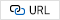3
Sargisson L., 2012, Fool’s Gold?: Utopianism in the Twenty-First Century, Springer, ISBN 9781137031075, Google describes Emporis.com as the first global provider of building data, the world’s database for buildings.4
Lissa Paulo, Peretti Correa Dayanne, Schukat Michael, Barrett Enda, Seri Federico, Keane Marcus, 2019, Machine Learning Methods Applied to Building Energy Production and Consumption Prediction.5
Gonzlez-Briones A., Hernández G., Corchado J. M., Omatu S., Mohamad M. S., 2019, Machine Learning Models for Electricity Consumption Forecasting: A Review, International Conference on Computer Applications & Information Security (ICCAIS)6
Klemenjak Christoph, Reinhardt Andreas, Pereira Lucas, Makonin Stephen, Bergs Mario, 2019, Electricity Consumption Data Sets: Pitfalls and Opportunities., pp. 159-1627
Li Zhang., 2020, Abnormal Energy Consumption Analysis Based on Big Data Mining Technology., pp. 64-688
Vantuch T., Vidal A. G., Ramallo-Gonzlez A. P., 2018, ,Machine learning based electric load forecasting for short and long-term period, 2018 IEEE 4th World Forum on Internet of Things (WF-IoT) 2018, pp. 511-5169
Ashouri Milad, Fung Benjamin, Haghighat Fariborz, Yoshino Hiroshi., 2019, Systematic Approach to Provide Building Occupants with Feedback to Reduce Energy Consumption., Energy. 194. 116813.10
Chengliang Xu , Huanxin Chen , 2020, ,A hybrid data mining approach for anomaly detection and evaluation in residential buildings energy data, Energy and Buildings, Volume 215, 15 May 2020, 10986411
Ullah A, Haydarov K, Ul Haq I, Muhammad K, Rho S, Lee M, Baik SW, 2020, Deep Learning Assisted Buildings Energy Consumption Profiling Using Smart Meter Data., Sensors. 20. 873. 10.3390/s20030873.12
Barber David, Cemgil A. Taylan, 2011, , Bayesian time series models., Cambridge University Press.13
Meuleau N., Peshkin L., Kim K.-E., 1999, , Learning finite state controllers for partially observable environments., In Proceedings of Fifteenth Conference on Uncertainty in Artificial Intelligence, pp. pages 427-43614
Ackley D. H., Hinton G. E., Sejnowski T. J., 1985, A Learning Algorithm for Boltzmann Machines., Cognitive Science, Vol. 9, pp. 147-16915
Hastie T., Friedman J., 2001, ,Boosting and Additive Trees., In: The Elements of Statistical Learning. Springer Series in Statistics. Springer, New York, NY.16
Madeh Piryonesi S., El-Diraby Tamer E., 2020, Data Analytics in Asset Management: Cost-Effective Prediction of the Pavement Condition Index., Journal of Infrastructure Systems. 26 (1): 04019036. ISSN 1943-555X.17
Pelleg Dan, Moore Andrew, 1999, Accelerating exact k-means algorithms with geometric reasoning., Proceedings of the Fifth ACM SIGKDD International Conference on Knowledge Discovery and Data Mining - KDD ’99. San Diego, California, United States: ACM Press: 277281.18
2021, The electricity consumption price of Electricity of Vietnam19
Chen Tianqi, Guestrin Carlos, XGBoost: A Scalable Tree Boosting System., In Krishnapuram, Balaji; Shah, Mohak; Smola, Alexander J.; Aggarwal, Charu C.; Shen, Dou; Rastogi, Rajeev (eds.). Proceedings of the 22nd ACM SIGKDD International Conference on Knowledge Discovery and Data Mining, San Francisco, CA, USA, August 13-17, 2016. ACM. pp. 785794.20
Hyndman Rob J, Athanasopoulos George., 8.9. Forecasting: principles and practice., texts. Retrieved 19 May 2015.21
Chen Z., Zhao YL., Pan XY., Dong ZY., Gao B., Zhong ZW., 2009, An Overview of Prophet., In: Hua A., Chang SL., eds) Algorithms and Architectures for Parallel Processing. ICA3PP 2009. Lecture Notes in Computer Science, vol 5574. Springer, Berlin, Heidelberg.Author

Nam Anh Dao received his B.S. in Applied Mathematics and a Ph.D. in Physics\textendash{}Mathematics from the University of Moldova in 1987 and 1992, respectively. He was involved in various international software projects. He is currently teaching at Electric Power University. His research interests include Intellectual Intelligence, Image Processing and Pattern Recognition, Machine Vision, and Data Science. Main works cover pattern recognition and image analysis, medical imaging, and machine learning with emphasis on computer vision. He has also served, or is currently serving, as a reviewer for many important Journals and Conferences in Image Processing and Pattern Recognition.

Hải Minh Nguyen is a sophomore undergraduate in Computer Science at Hanoi University of Science and Technology (HUST), Viet Nam. He was involved in power consumption prediction project. His research interests include Machine Learning and Quantitative Optimization.

Tung Nguyen Khanh received his B.S. in Computer Information Systems from Vietnam National University-University of Engineering and Technology (VNU-UET) in 2016. Currently, he is a researcher at the university. Before starting his graduate studies, he worked at Electricity Company of Vietnam (EVN) as a research engineer. He was involved in various projects, including building an IoT Secured SmartGateway for electric cabinets and developing an online Network Security Scanner. His research interests include electrical data mining and cybersecurity for electrical operation networks.